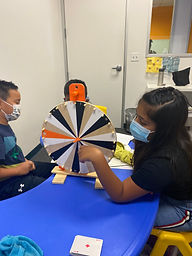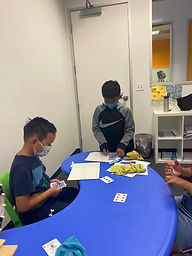## Ms. Briana

### Target 1​

###### Lesson Type:

New

Number Operation

:

Number Classes

Understand that a whole number can be described as even or odd.

###### 1:

Find all the even and odd numbers on a hundred’s chart.

###### 2:

Highlight the pattern that occurs in the number sequence with even and odd numbers (even, odd, even, odd, even…).

###### 3:

Understand that the digit in the ones’ place determines whether a number is even or odd.

###### 4:

Recognize that even numbers have a 0, 2, 4, 6, or 8 in the ones’ place.

###### 5:

Recognize that odd numbers have a 1, 3, 5, 7, or 9 in the ones’ place.

2nd

###### Vocabulary:

Even, Odd and Sequence

Activities:

Students color coded even and odd number 1-100.

Students used dice to create different quantities for them to classify as either even or odd.

Students used a large spinning wheel to create quantities to be classified.### Home Exploration

###### Guiding Questions:## Absent Students:

### Target 2

:

###### 1:

Identify the pattern that occurs when two even numbers are added.

###### 2:

Identify the pattern that occurs when two odd numbers are added.

###### 3:

Identify the pattern that occurs when an even and odd number are added.

2nd

###### Vocabulary:

Even, Odd, Sum and Sequence

Activities:

Students created addition problems using playing cards where they added 2 even quantities.

Students then created addition problems using playing cards where they added 2 odd quantities.

Students then created addition problems using playing cards where they added 1 even and 1 odd quantity.

Students then were able to recognize the pattern and identify the sum results withut solving.### Home Exploration

###### Guiding Questions:### Target 3

:

###### 1:

Understand that dividing numbers makes numbers smaller.

###### 2:

Understand that division equations can be written both vertically and horizontally and division can be indicated using multiple symbols (i.e., ÷, ⟌).

###### 3:

Understand that division can be represented with repeated subtraction.

3rd

###### Vocabulary:

Dividing, Quantity, Repeated Subtraction

Activities:

Students used playing cards to create two and three digit quanities to divide by single intergers.

Students practices solving basic division problems using repeated subtraction on whiteboards.### Home Exploration